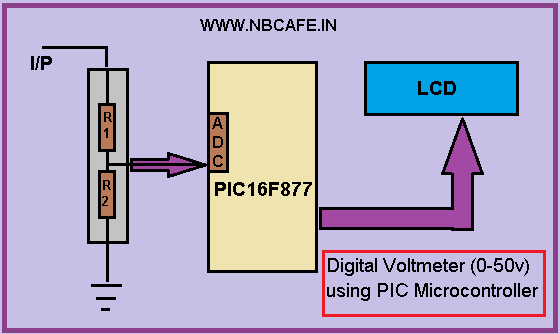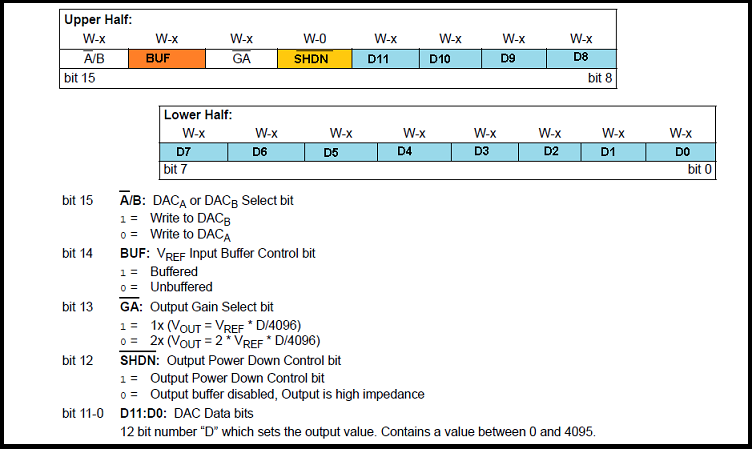# Digital Voltmeter (0-50v) using PIC Microcontroller

If we know how inbuilt ADC work then we can easily build Digital Voltmeter using PIC Microcontroller. In my previous post, we can see how easily we build “Digital thermometer with auto saving log file in excel by Pic microcontroller”. Using the same principle here we build Digital Voltmeter using PIC Microcontroller.

Requirement:To make digital voltmeter we uses some material here I give you the list of that.

1)    PIC16F877

2)    LCD (lm106l)

3)    Resistors (100k & 11.11k).

Project Details:

In this project we use internal ADC of PIC16f877 microcontroller for getting input voltage which we have to measure. After getting the input it processed in microcontroller and the equivalent voltage is display in the LCD which is attached with port D. In bellow see the block diagram of our project Digital Voltmeter using PIC Microcontroller.

Working Principal:

We know that PIC16f877 has 10 bit ADC. So input in form 10 bit and we need 8 bit data to farther process. So getting equivalent integer value we have to convert the 10 bit ADC value in equivalent integer number. Now question is how we convert it? For converting it we have to take a reference voltage let we take reference voltage 5 volt. Now the formula of conversion is

Value =value * (reference millivolt / 210-1)

=value *  5000/1024-1

= value * 4.89

Now here we can use maximum reference voltage 5 Volt beyond that voltage PIC cannot tolerate. Now if we give max 5v as reference then how can we measure 50v? We cannot give 50v at input pin of in build ADC for measure 50 v DC. So we have to reduce the voltage with proportionate with reference voltage 5v. For that reason here we introduce Voltage divider circuit with taking R1 = 100k and R2 = 11.11k. Now for selecting R1 and R2 we use voltage divider formula I,e

READ  Wireless MultiMeter using PIC18F452 Microcontroller

Vout = (R2/R1+R2) * Vin .

So using above formula let see what happen if we want to measure 50v

Vout = (11.11/100+11.11)*50

= 5v

So we can easily measure maximum 50 v DC. See the circuit diagram for proteus simulation.

For more detail: Digital Voltmeter (0-50v) using PIC Microcontroller

#### Current Project / Post can also be found using:

• pic voltmeter 7 segment
• voltmeter diagram microchip
• pic16f676 voltmeter seven segment
• pic voltmeter with pic16f676